Ex 5.4

Chapter 5 Class 6 Understanding Elementary Shapes
Serial order wise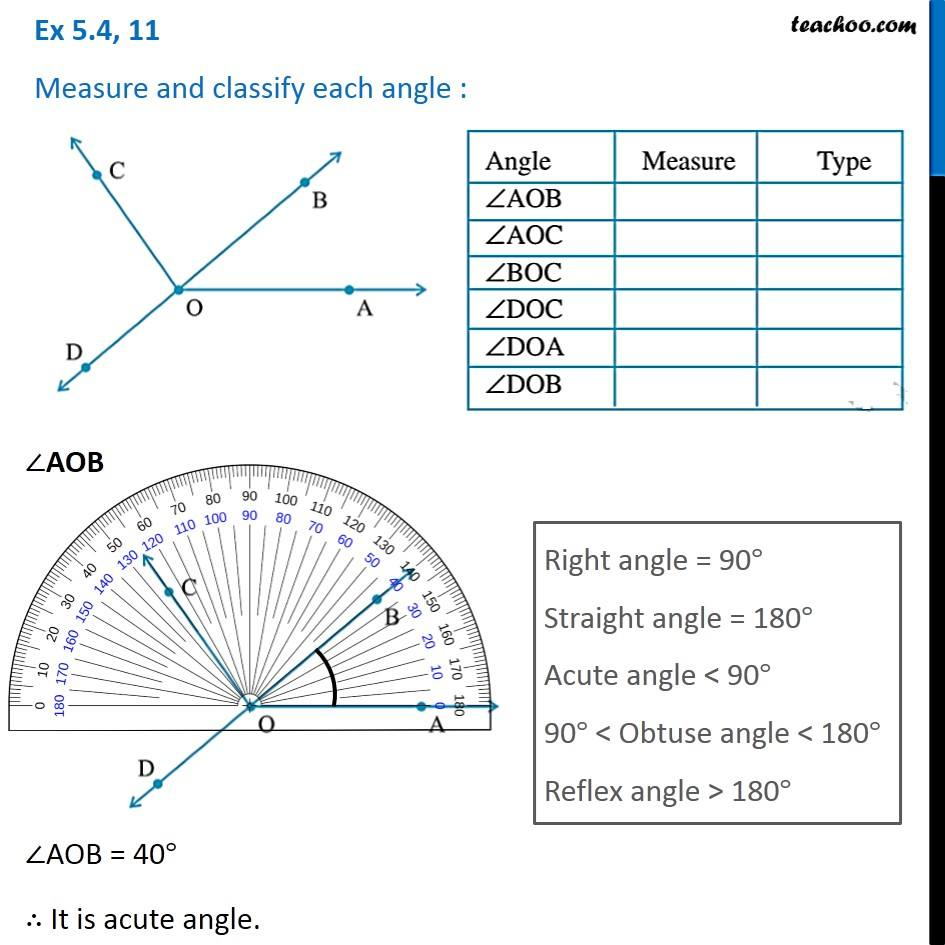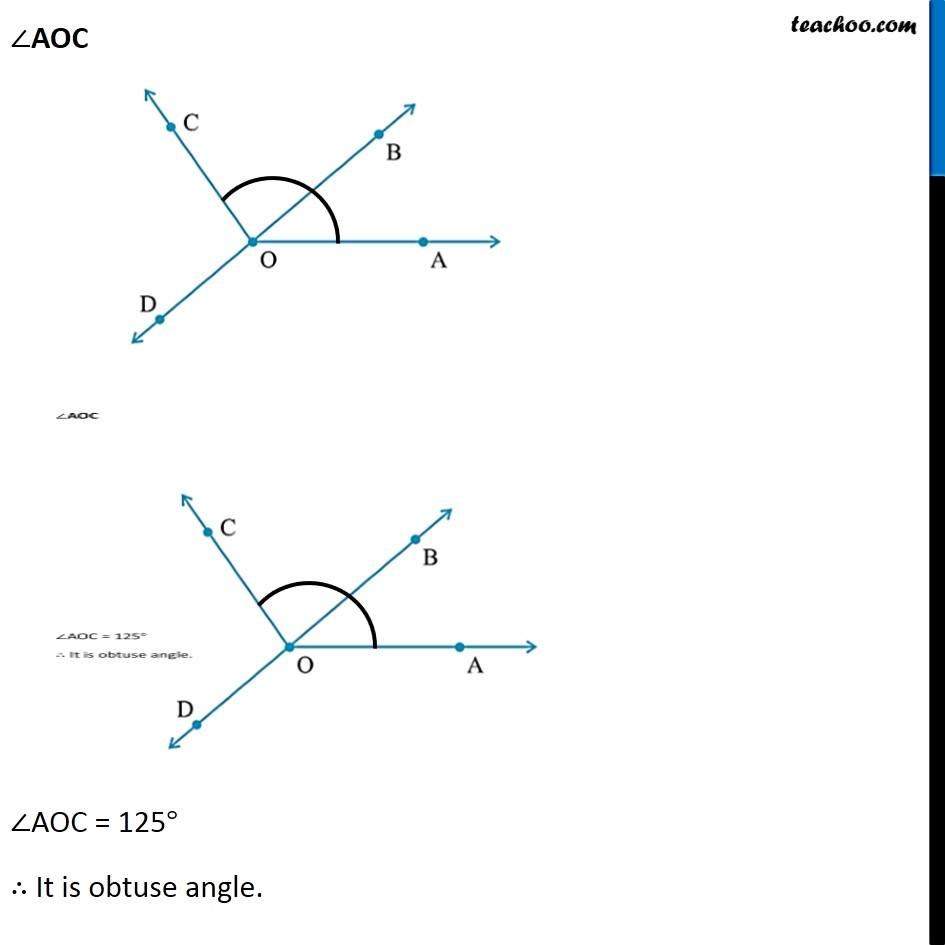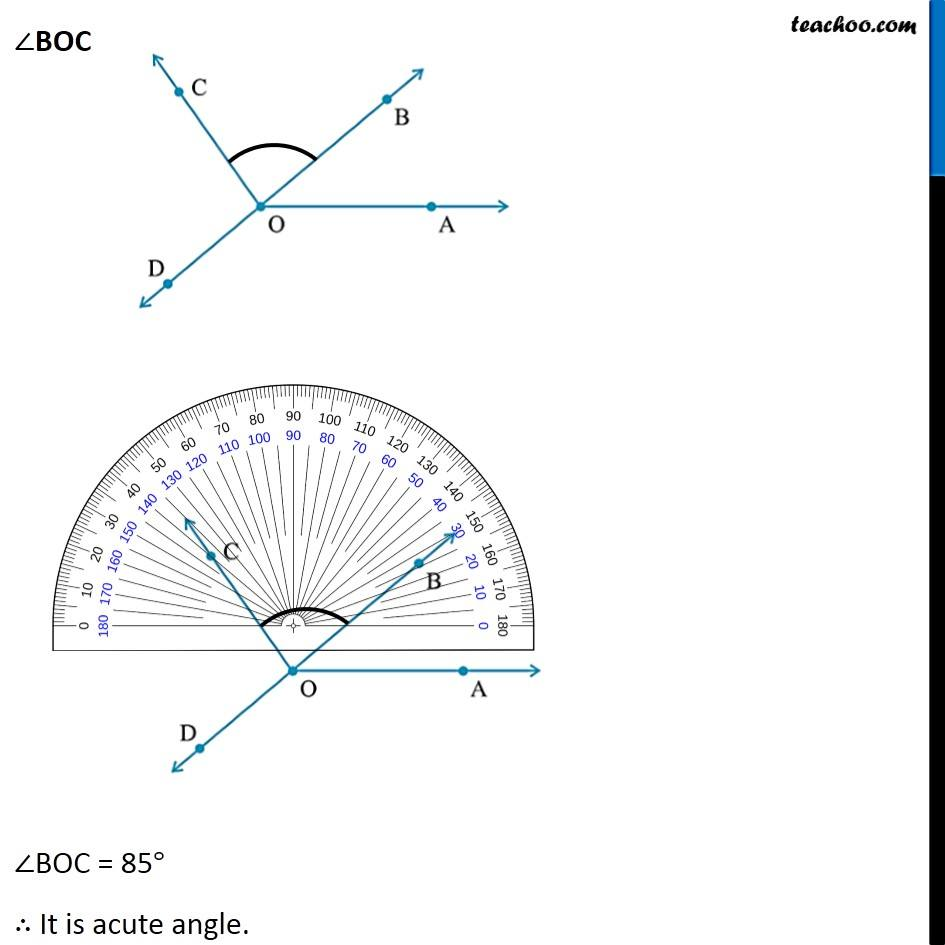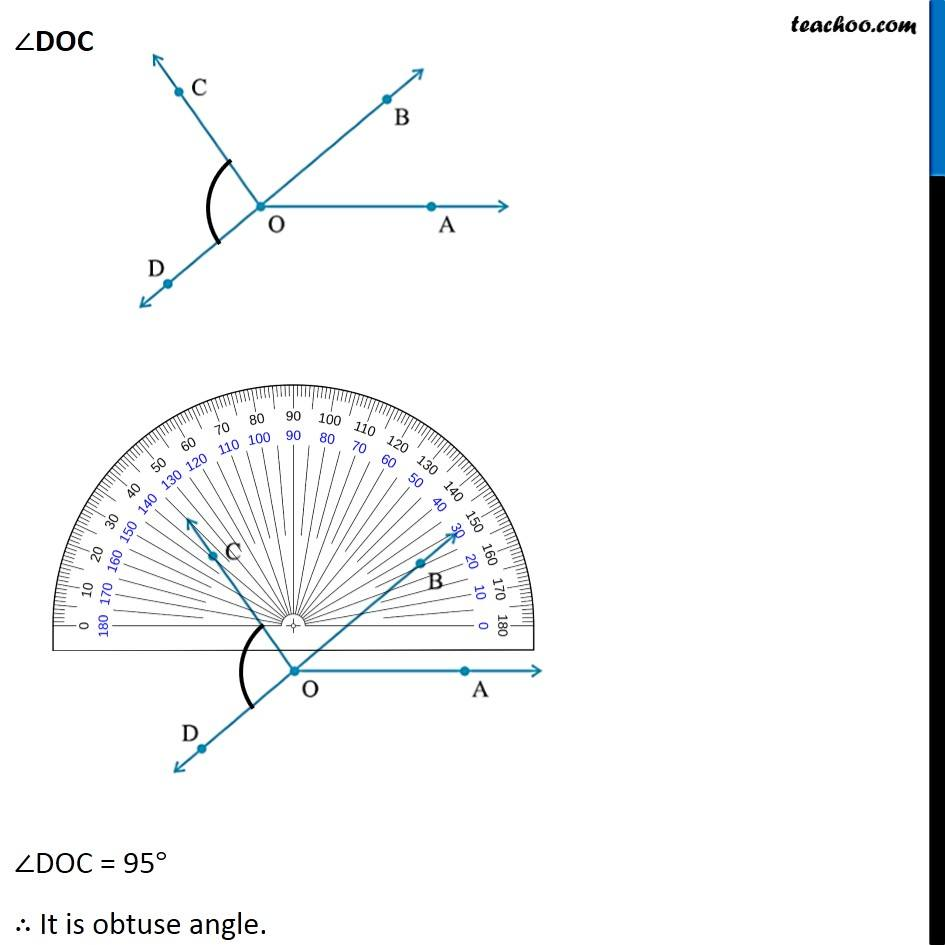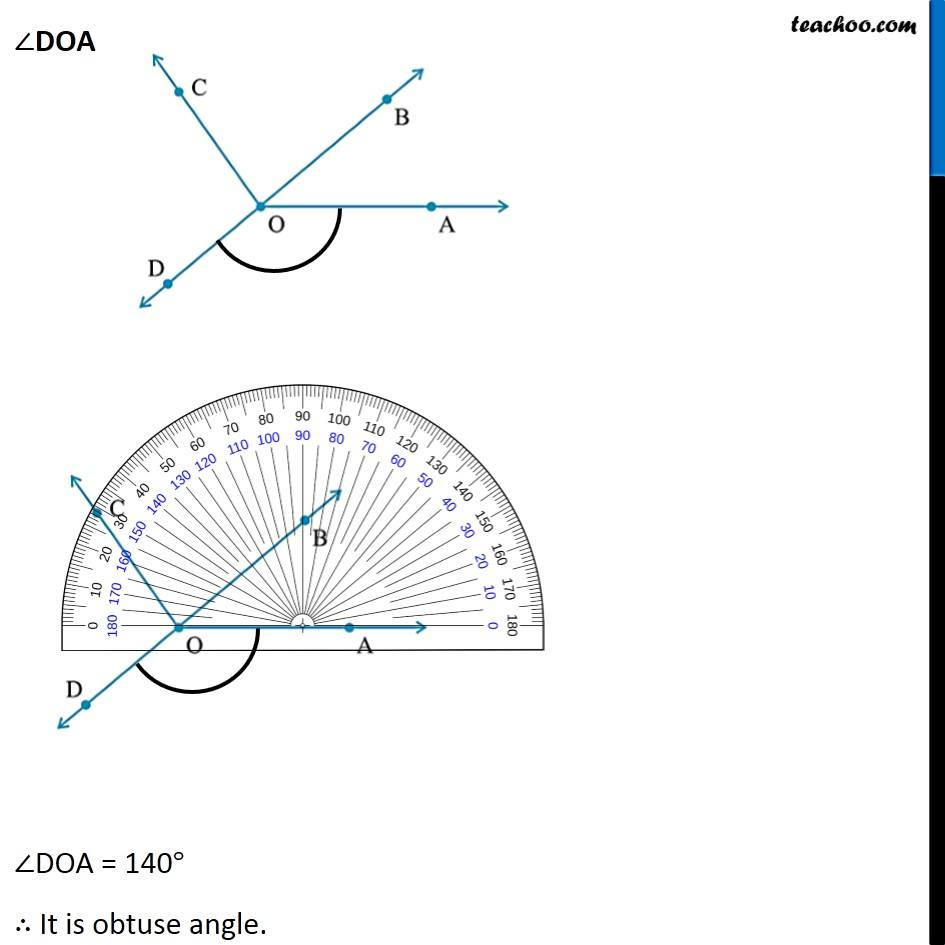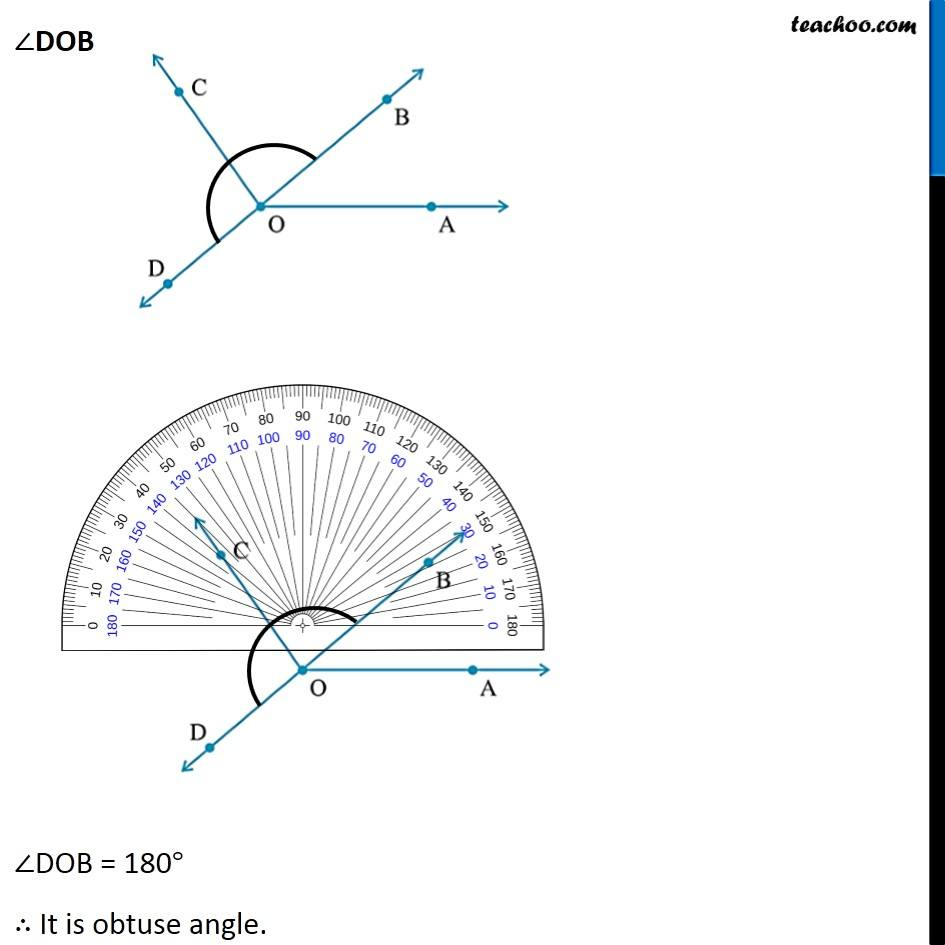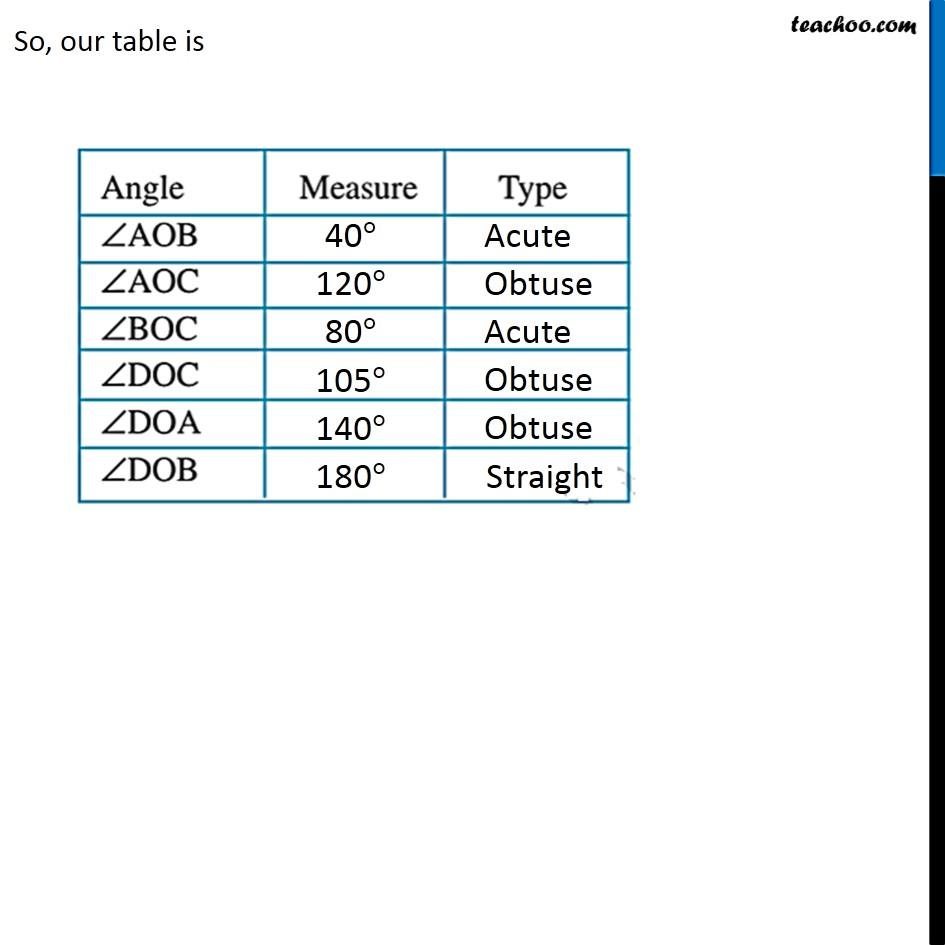Maths Crash Course - Live lectures + all videos + Real time Doubt solving!

### Transcript

Ex 5.4, 11 Measure and classify each angle : ∠AOB ∠AOB = 40° ∴ It is acute angle. ∠AOC ∠AOC = 125° ∴ It is obtuse angle. ∠BOC ∠BOC = 85° ∴ It is acute angle. ∠DOC ∠DOC = 95° ∴ It is obtuse angle. ∠DOA ∠DOA = 140° ∴ It is obtuse angle. ∠DOB ∠DOB = 180° ∴ It is obtuse angle. So, our table is